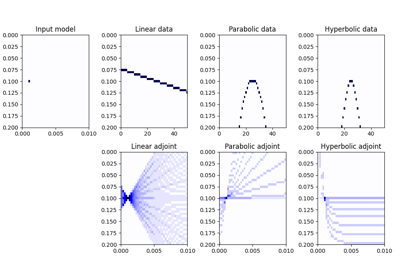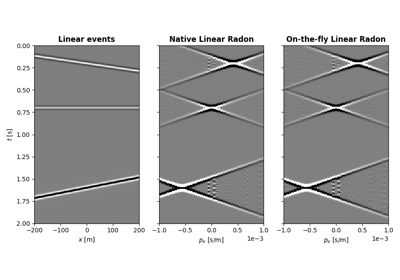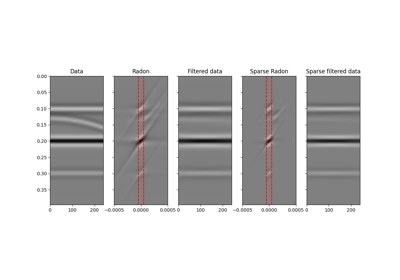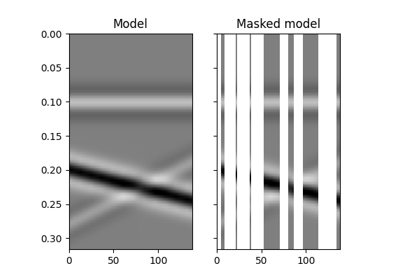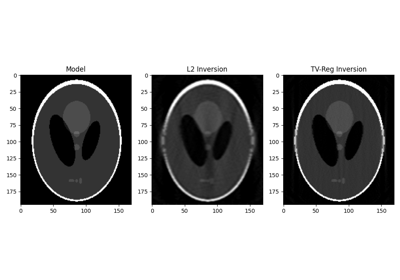class pylops.Spread(dims, dimsd, table=None, dtable=None, fh=None, interp=None, engine='numpy', dtype='float64')[source]

Spread values from the input model vector arranged as a 2-dimensional array of size $$[n_{x_0} \times n_{t_0}]$$ into the data vector of size $$[n_x \times n_t]$$. Note that the value at each single pair $$(x_0, t_0)$$ in the input is spread over the entire $$x$$ axis in the output.

Spreading is performed along parametric curves provided as look-up table of pre-computed indices (table) or computed on-the-fly using a function handle (fh).

In adjont mode, values from the data vector are instead stacked along the same parametric curves.

Parameters: dims : tuple Dimensions of model vector (vector will be reshaped internally into a two-dimensional array of size $$[n_{x_0} \times n_{t_0}]$$, where the first dimension is the spreading direction) dimsd : tuple Dimensions of data vector (vector will be reshaped internal into a two-dimensional array of size $$[n_x \times n_t]$$, where the first dimension is the stacking direction) table : np.ndarray, optional Look-up table of indices of size $$[n_{x_0} \times n_{t_0} \times n_x]$$ (if None use function handle fh). When dtable is not provided, the data will be created as follows data[ix, table[ix0, it0, ix]] += model[ix0, it0]  Note When using table without dtable, its elements must be between 0 and $$n_{t_0} - 1$$ (or numpy.nan). dtable : np.ndarray, optional Look-up table of decimals remainders for linear interpolation of size $$[n_{x_0} \times n_{t_0} \times n_x]$$ (if None use function handle fh). When provided, the data will be created as follows data[ix, table[ix0, it0, ix]] += (1 - dtable[ix0, it0, ix]) * model[ix0, it0] data[ix, table[ix0, it0, ix] + 1] += dtable[ix0, it0, ix] * model[ix0, it0]  Note When using table and dtable, the elements of table indices must be between 0 and $$n_{t_0} - 2$$ (or numpy.nan). fh : callable, optional If None will use look-up table table. When provided, should be a function which takes indices ix0 and it0 and returns an array of size $$n_x$$ containing each respective time index. Alternatively, if linear interpolation is required, it should output in addition to the time indices, a weight for interpolation with linear interpolation, to be used as follows data[ix, index] += (1 - dindices[ix]) * model[ix0, it0] data[ix, index + 1] += dindices[ix] * model[ix0, it0]  where index refers to a time index in the first array returned by fh and dindices refers to the weight in the second array returned by fh. Note When using fh with one output (time indices), the time indices must be between 0 and $$n_{t_0} - 1$$ (or numpy.nan). When using fh with two outputs (time indices and weights), they must be within the between 0 and $$n_{t_0} - 2$$ (or numpy.nan). interp : bool, optional Use only if engine engine='numba'. Apply linear interpolation (True) or nearest interpolation (False) during stacking/spreading along parametric curve. When using engine="numpy", it will be inferred directly from fh or the presence of dtable. engine : str, optional Engine used for spread computation (numpy or numba). Note that numba can only be used when providing a look-up table dtype : str, optional Type of elements in input array. KeyError If engine is neither numpy nor numba NotImplementedError If both table and fh are not provided ValueError If table has shape different from $$[n_{x_0} \times n_{t_0} \times n_x]$$

Notes

The Spread operator applies the following linear transform in forward mode to the model vector after reshaping it into a 2-dimensional array of size $$[n_x \times n_t]$$:

$m(x_0, t_0) \rightarrow d(x, t=f(x_0, x, t_0)) \quad \forall x$

where $$f(x_0, x, t)$$ is a mapping function that returns a value $$t$$ given values $$x_0$$, $$x$$, and $$t_0$$. Note that for each $$(x_0, t_0)$$ pair, spreading is done over the entire $$x$$ axis in the data domain.

In adjoint mode, the model is reconstructed by means of the following stacking operation:

$m(x_0, t_0) = \int{d(x, t=f(x_0, x, t_0))} \,\mathrm{d}x$

Note that table (or fh) must return integer numbers representing indices in the axis $$t$$. However it also possible to perform linear interpolation as part of the spreading/stacking process by providing the decimal part of the mapping function ($$t - \lfloor t \rfloor$$) either in dtable input parameter or as second value in the return of fh function.

Attributes: shape : tuple Operator shape explicit : bool Operator contains a matrix that can be solved explicitly (True) or not (False)

Methods

 __init__(dims, dimsd[, table, dtable, fh, …]) Initialize this LinearOperator. adjoint() Hermitian adjoint. apply_columns(cols) Apply subset of columns of operator cond([uselobpcg]) Condition number of linear operator. conj() Complex conjugate operator div(y[, niter, densesolver]) Solve the linear problem $$\mathbf{y}=\mathbf{A}\mathbf{x}$$. dot(x) Matrix-matrix or matrix-vector multiplication. eigs([neigs, symmetric, niter, uselobpcg]) Most significant eigenvalues of linear operator. matmat(X) Matrix-matrix multiplication. matvec(x) Matrix-vector multiplication. rmatmat(X) Matrix-matrix multiplication. rmatvec(x) Adjoint matrix-vector multiplication. todense([backend]) Return dense matrix. toimag([forw, adj]) Imag operator toreal([forw, adj]) Real operator tosparse() Return sparse matrix. trace([neval, method, backend]) Trace of linear operator. transpose() Transpose this linear operator.

## Examples using pylops.Spread¶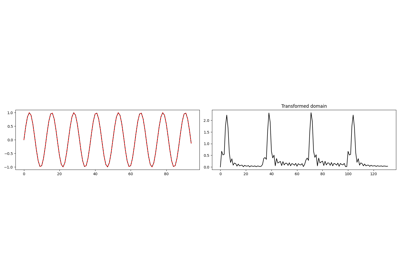1D, 2D and 3D Sliding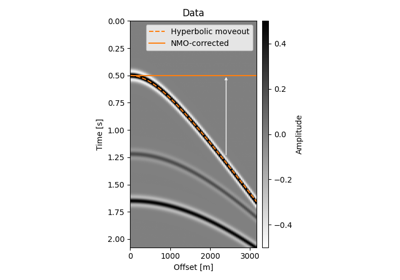Normal Moveout (NMO) Correction